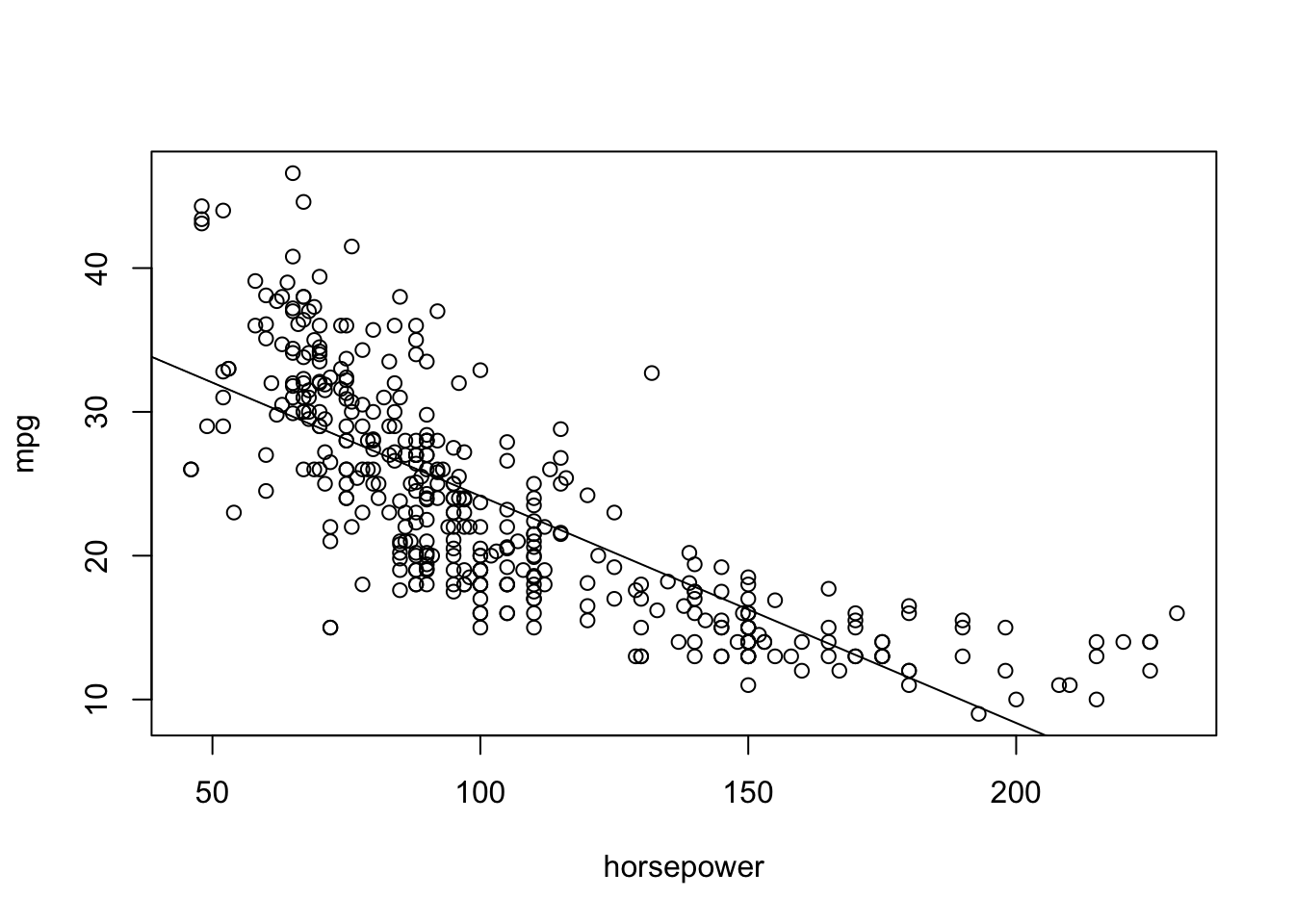ISLR Home

# Question

p121

This question involves the use of simple linear regression on the Auto data set.

1. Use the lm() function to perform a simple linear regression with mpg as the response and horsepower as the predictor. Use the summary() function to print the results. Comment on the output. For example
1. Is there a relationship between the predictor and the response?
2. How strong is the relationship between the predictor and the response?
3. Is the relationship between the predictor and the response positive or negative?
4. What is the predicted mpg associated with a horsepower of 98? What are the associated 95 % confidence and prediction intervals?
1. Plot the response and the predictor. Use the abline() function to display the least squares regression line.

2. Use the plot() function to produce diagnostic plots of the least squares regression fit. Comment on any problems you see with the fit.

``````library(MASS)
library(ISLR)``````

# 8a Simple linear regression model

Name of the columns

``names(Auto)``
``````##  "mpg"          "cylinders"    "displacement" "horsepower"   "weight"
##  "acceleration" "year"         "origin"       "name"``````

## Fit Model: mpg ~ horsepower

``auto.lm = lm(mpg ~ horsepower, data=Auto)``

## Model Summary

``summary(auto.lm)``
``````##
## Call:
## lm(formula = mpg ~ horsepower, data = Auto)
##
## Residuals:
##      Min       1Q   Median       3Q      Max
## -13.5710  -3.2592  -0.3435   2.7630  16.9240
##
## Coefficients:
##              Estimate Std. Error t value Pr(>|t|)
## (Intercept) 39.935861   0.717499   55.66   <2e-16 ***
## horsepower  -0.157845   0.006446  -24.49   <2e-16 ***
## ---
## Signif. codes:  0 '***' 0.001 '**' 0.01 '*' 0.05 '.' 0.1 ' ' 1
##
## Residual standard error: 4.906 on 390 degrees of freedom
## Multiple R-squared:  0.6059, Adjusted R-squared:  0.6049
## F-statistic: 599.7 on 1 and 390 DF,  p-value: < 2.2e-16``````

## i. Is there a relationship between the predictor and the response?

1. (Answers obtained using summary(auto.lm)) There is a relationship between horsepower (predictor) and mpg (response) because the p-value is extremely below 0.05, which means that chances that this relationship occurred, when there is no relationship at all , is extremely slim, therefore there has to be a relationship

## ii. How strong is the relationship between the predictor and the response?

1. (Answers obtained using summary(auto.lm)) The relationship is strong, about 60%, because the R^2 = .6059. This statistic measures the proportion of variability in response that can be explained using the predictor.

## iii. Is the relationship between the predictor and the response positive or negative?

1. (Answers obtained using summary(auto.lm)) The relationship between mpg and horsepower has a negative relationship because the coefficient of horsepower (predictor) is negative

1. ## Predict

``predict(auto.lm, data.frame(horsepower=c(98)), interval="prediction")``
``````##        fit     lwr      upr
## 1 24.46708 14.8094 34.12476``````

# 8b Plot Regression Line

1. Plot the response and the predictor. Use the abline() function to display the least squares regression line.
``````attach(Auto)
plot(horsepower, mpg) # Plot points
abline(auto.lm) # Add Least Squares Regression Line``````# 8c Diagnostic Plots

1. Use the plot() function to produce diagnostic plots of the least squares regression fit. Comment on any problems you see with the fit.
``````par(mfrow = c(2,2)) # 4 plots in same picture
plot(auto.lm)``````